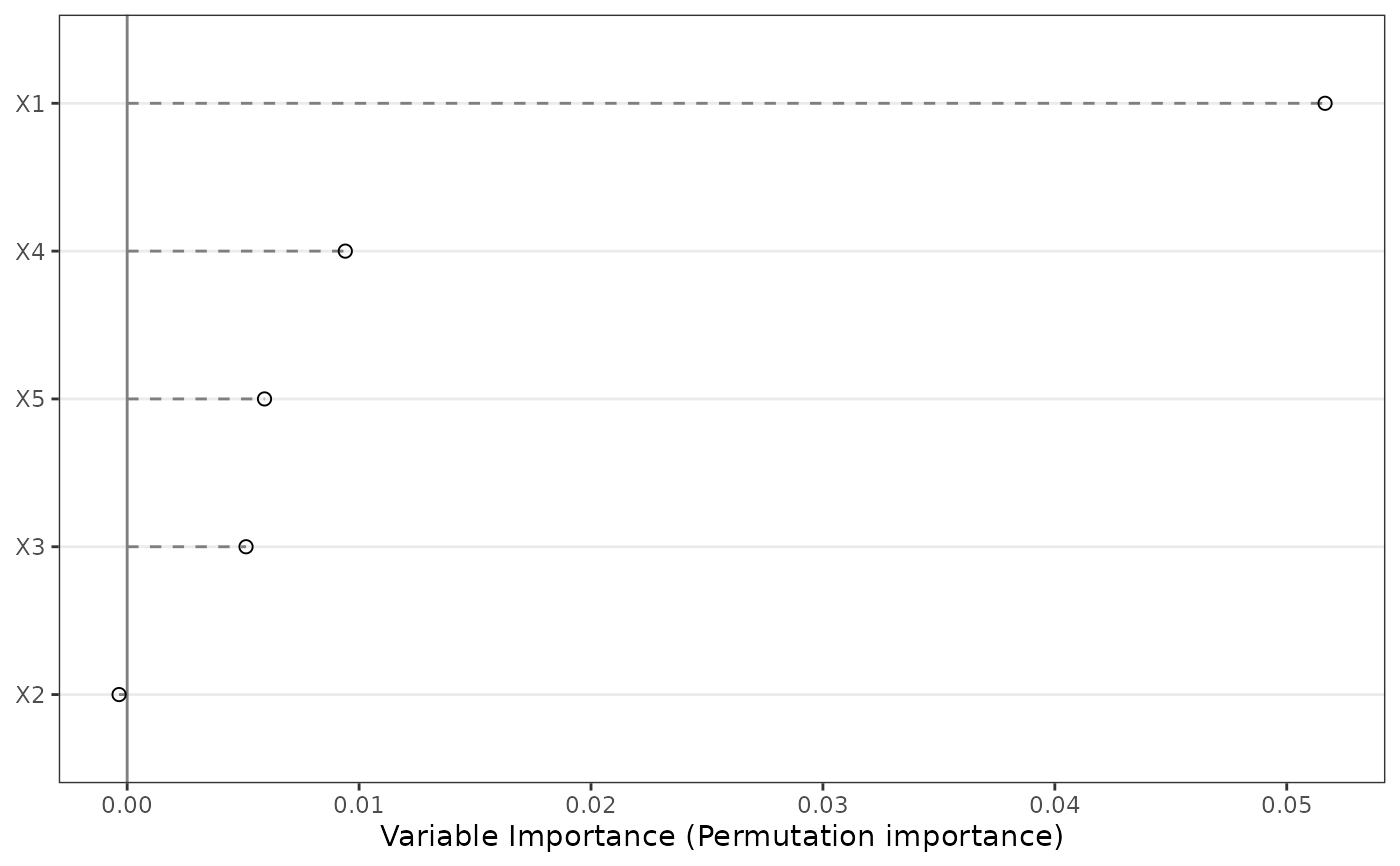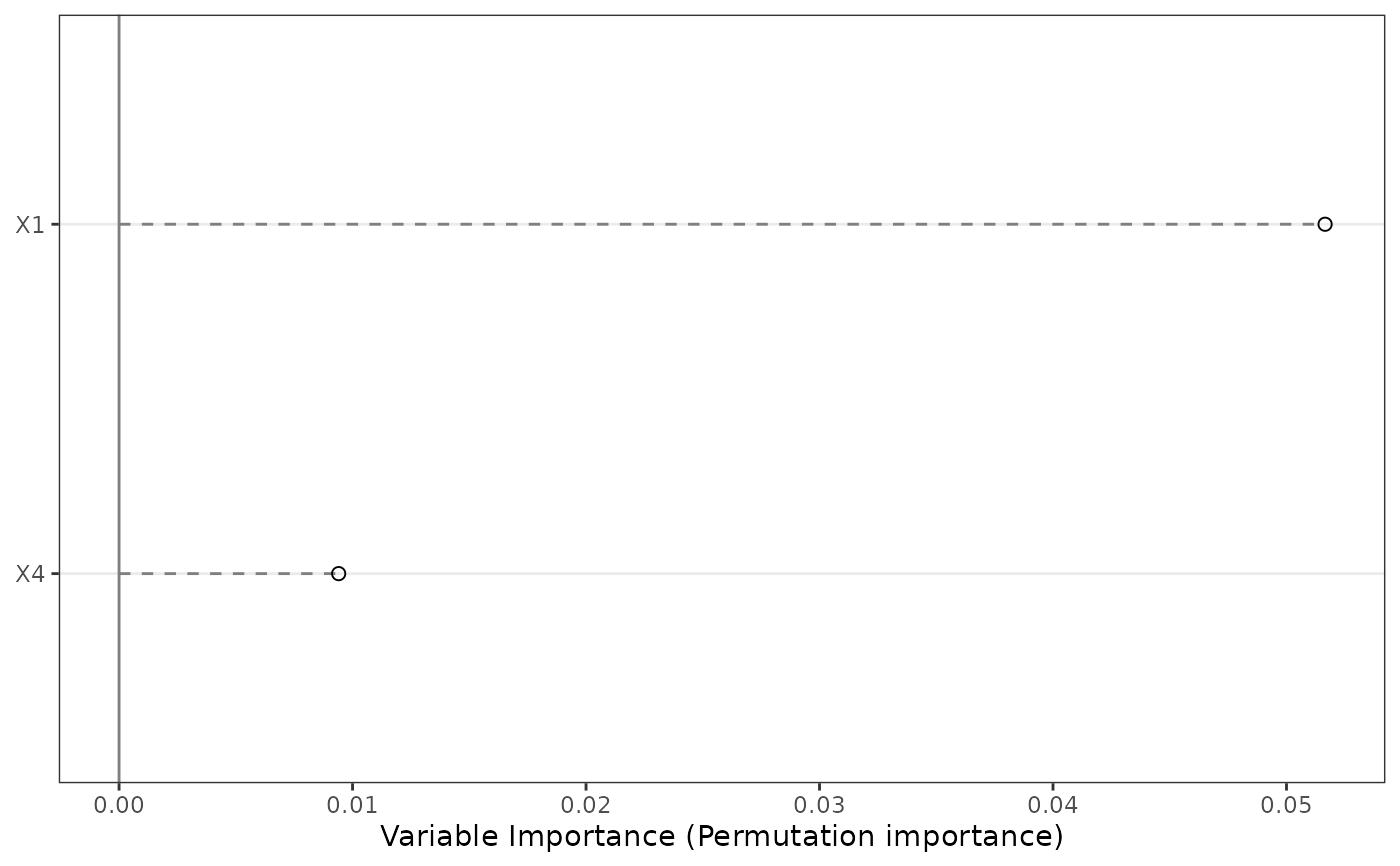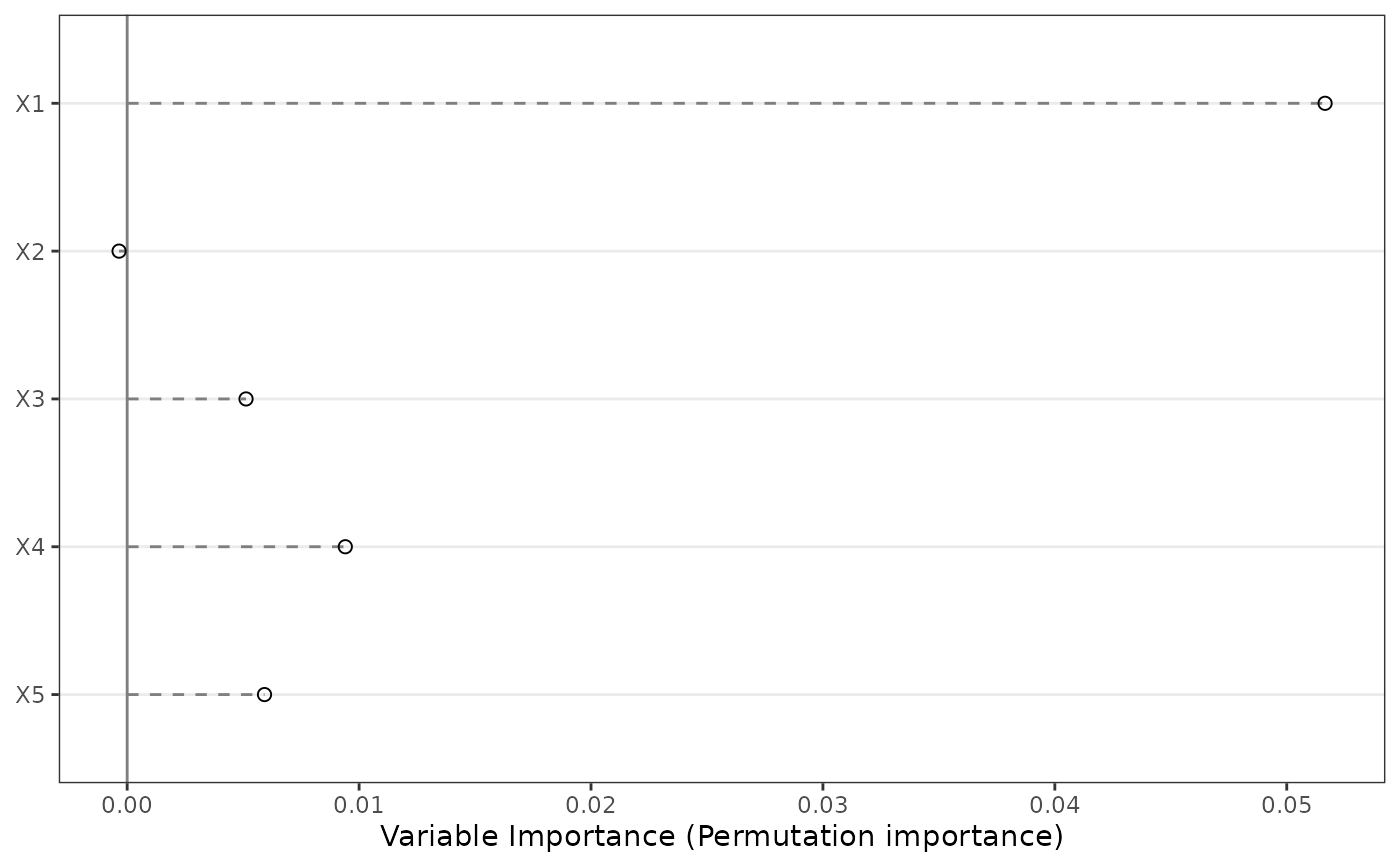Plots variable importance for a MetaForest object.

VarImpPlot(mf, n.var = 30, sort = TRUE, ...)

## Arguments

mf MetaForest object. Number of moderators to plot. Should the moderators be sorted from most to least important? Parameters passed to and from other functions.

A ggplot object.

## Examples

set.seed(42)
data <- SimulateSMD()
mf.random <- MetaForest(formula = yi ~ ., data = data\$training,
whichweights = "random", method = "DL",
tau2 = 0.0116)
VarImpPlot(mf.random)VarImpPlot(mf.random, n.var = 2)VarImpPlot(mf.random, sort = FALSE)